##全国校区# 分析5万多场英雄联盟比赛，教你如何轻松用python预测胜负2020-10-27CDA数据分析师 出品

【导读】01、项目介绍

• Towers（防御塔）：每支队伍总共有11座防御塔
• Inhibitor（水晶）：每条道有一个水晶
• Elemental Drakes/Elder Dragon（大龙/远古龙）
• Rift Herald（峡谷先锋）
• Baron Nasho（纳什男爵）
• Nexus（基地）

02、数据集概述

• Game ID：游戏ID
• Creation Time：创建时间
• Game Duration (in seconds)：游戏持续时间（秒）
• Season ID：赛季ID
• Winner (1=team1, 2=team2)：获胜队伍
• First Baron, dragon, tower, blood, inhibitor and Rift Herald (1 = team1, 2 = team2, 0 = none)：第一条纳什男爵，大龙，塔，一血，水晶，峡谷先锋
• Champions and summoner spells for each team (Stored as Riot's champion and summoner spell IDs)：每只队伍选择的英雄和召唤术
• The number of tower, inhibitor, Baron, dragon and Rift Herald kills each team has：塔，水晶，男爵，大龙和峡谷先锋击杀数
• The 5 bans of each team (Again, champion IDs are used)：每个队伍的禁用英雄

03、数据读入和预览

```# 数据整理
import numpy as np
import pandas as pd

# 可视化
import matplotlib.pyplot as plt
import seaborn as sns
import plotly as py
import plotly.graph_objs as go

# 建模
from sklearn.tree import DecisionTreeClassifier
from sklearn.model_selection import train_test_split, GridSearchCV
from sklearn.metrics import confusion_matrix, classification_report ```
```# 读入数据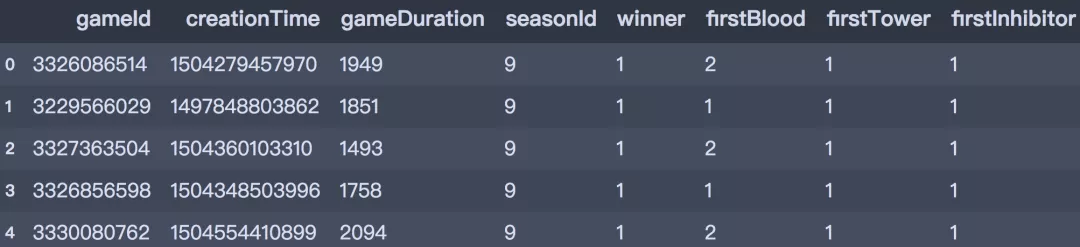```df.shape
(51490, 61)```

04、数据可视化

1. 目标变量分布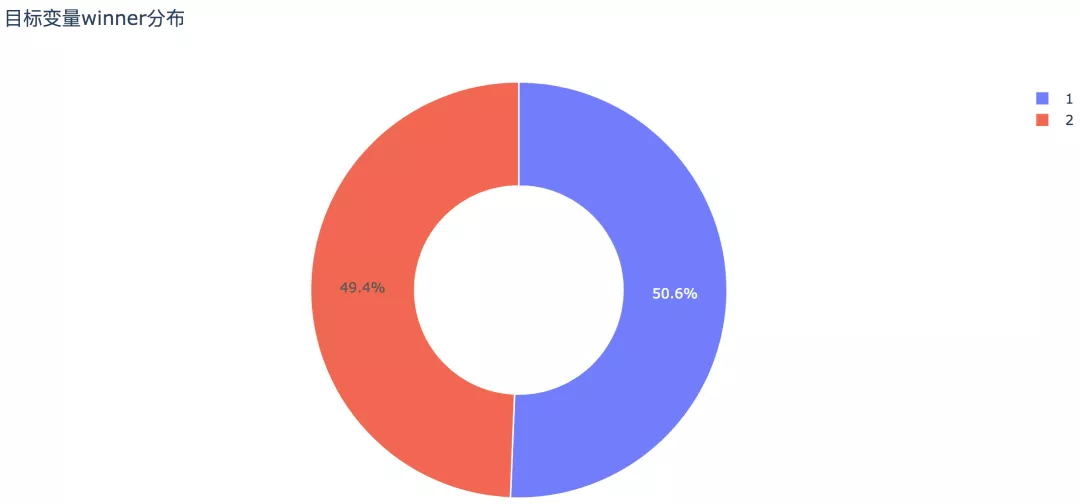——代码如下：

```# 饼图
trace0 = go.Pie(labels=df['winner'].value_counts().index,
values=df['winner'].value_counts().values,
hole=0.5,
opacity=0.9,
marker=dict(line=dict(color='white', width=1.3))
)
layout = go.Layout(title='目标变量winner分布')

data = [trace0]
fig = go.Figure(data, layout)
py.offline.plot(fig, filename='./html/整体获胜情况分布.html')```

2. 游戏时长分布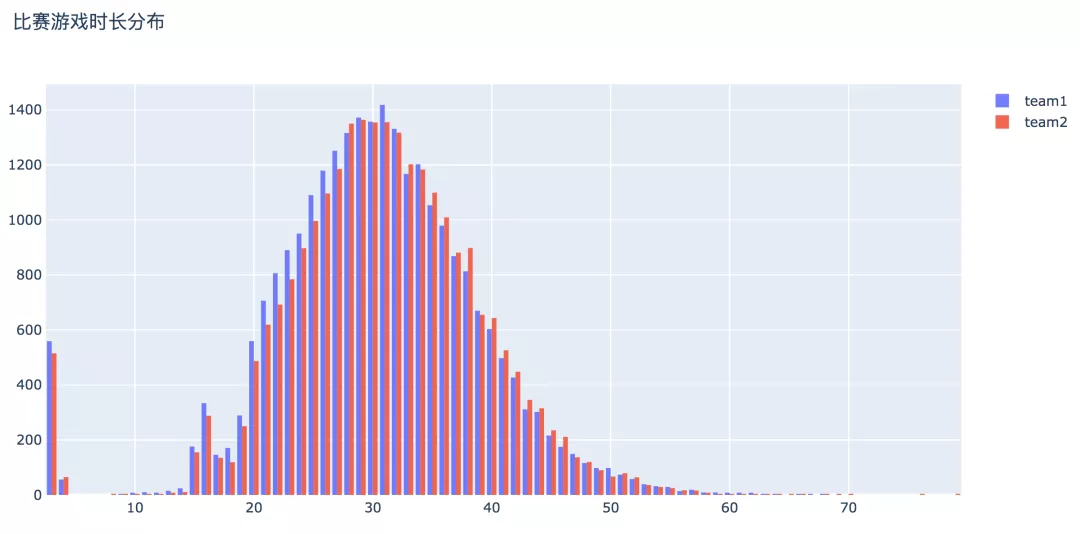——代码：

```df['game_duration'] = round(df['gameDuration'] / 60)

# 选择数据
x1 = df[df['winner'] == 1]['game_duration']
x2 = df[df['winner'] == 2]['game_duration']

# 直方图
trace0 = go.Histogram(x=x1, bingroup=25, name='team1', opacity=0.9)
trace1 = go.Histogram(x=x2, bingroup=25, name='team2', opacity=0.9)

layout = go.Layout(title='比赛游戏时长分布')

data = [trace0, trace1]
fig = go.Figure(data, layout)
py.offline.plot(fig, filename='./html/游戏时长分布.html')```

3. 一血对获胜的影响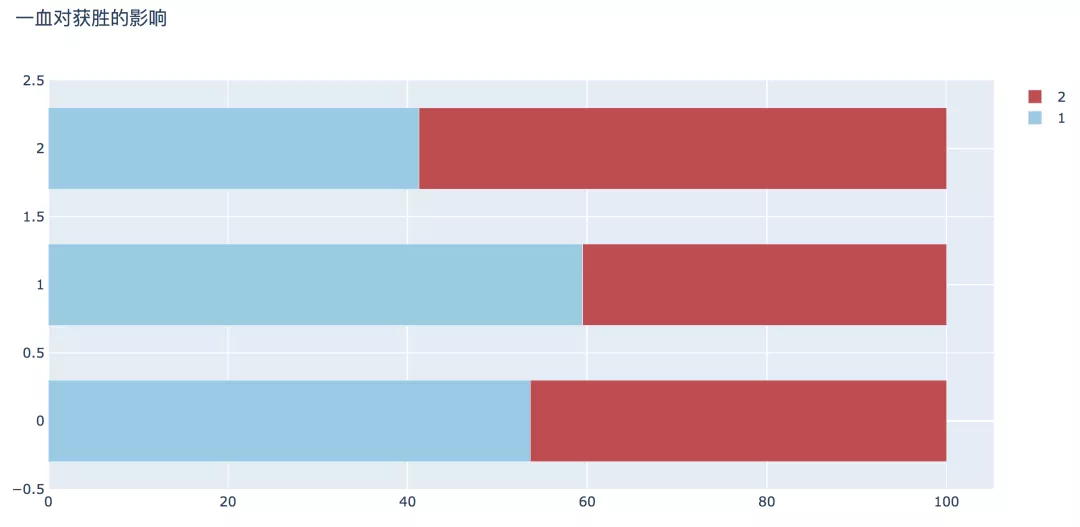——代码为：

```plot_bar_horizontal(input_col='firstBlood',
target_col='winner',
title_name='一血对获胜的影响')  ```

4. 一塔对获胜的影响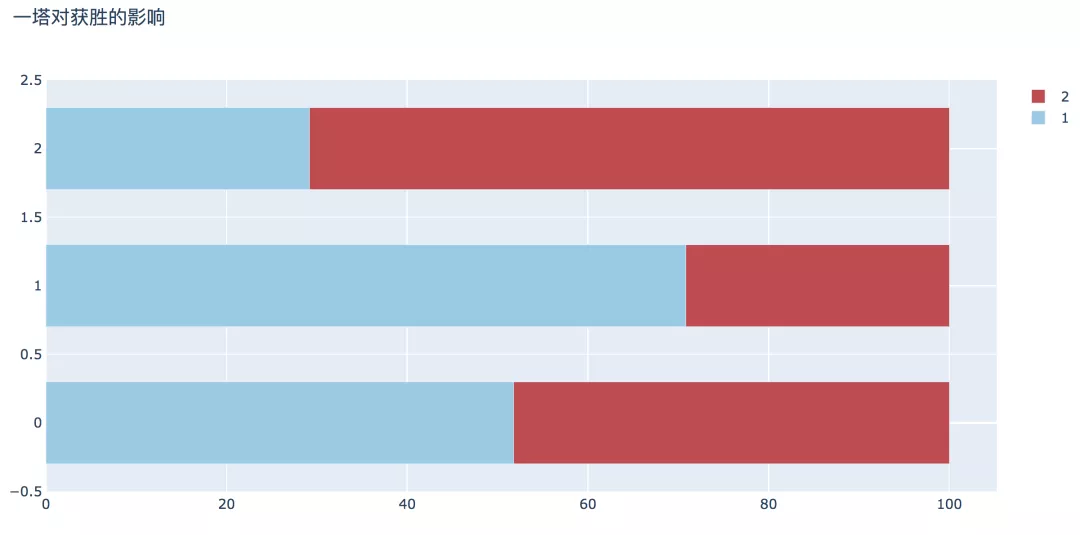——代码是：

```plot_bar_horizontal(input_col='firstTower',
target_col='winner',
title_name='一塔对获胜的影响')   ```

5. 摧毁第一个水晶对获胜的影响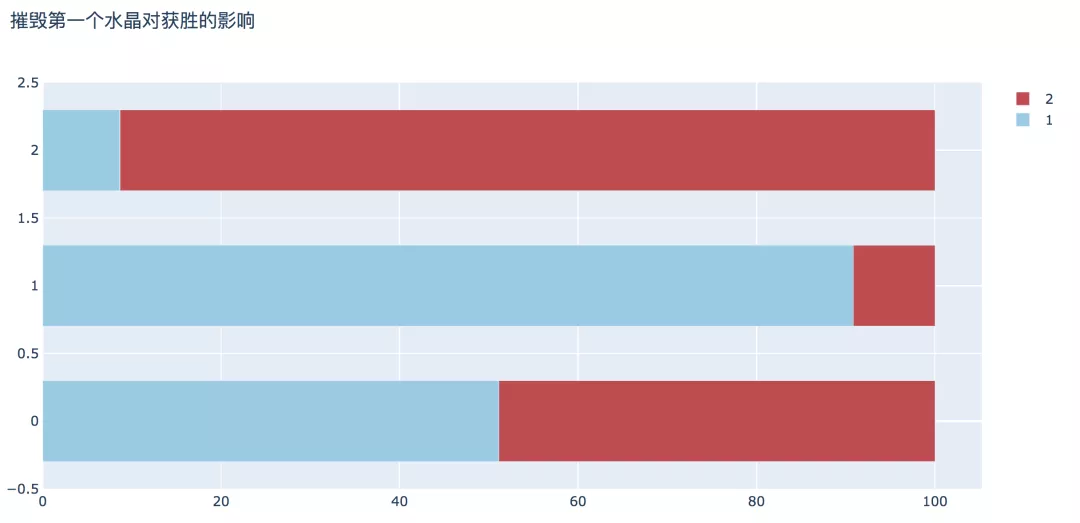——代码为：

```plot_bar_horizontal(input_col='firstInhibitor',
target_col='winner',
title_name='摧毁第一个水晶对获胜的影响')     ```

6. 击杀第一条男爵对获胜影响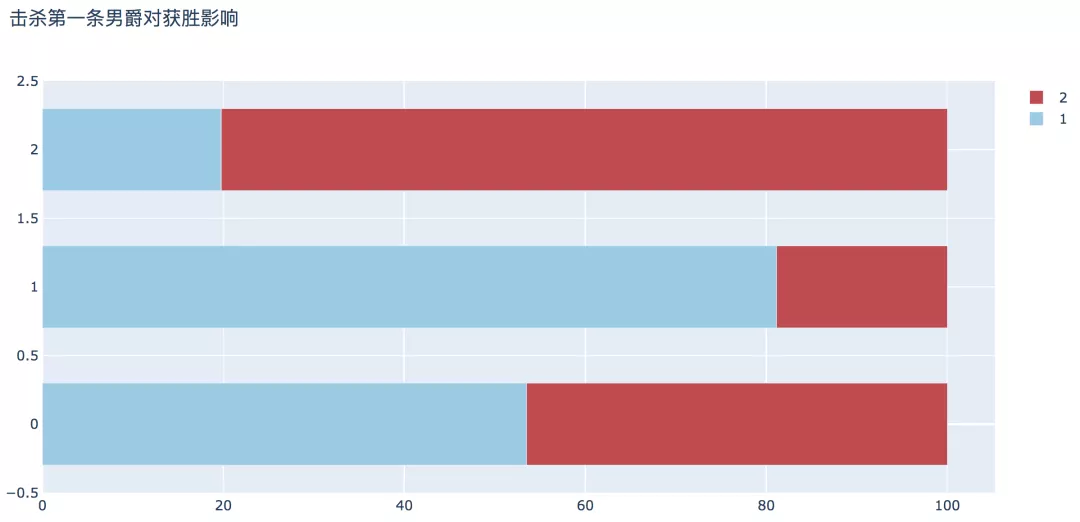```plot_bar_horizontal(input_col='firstBaron',
target_col='winner',
title_name='击杀第一条男爵对获胜影响')   ```

7. 击杀第一条大龙对获胜的影响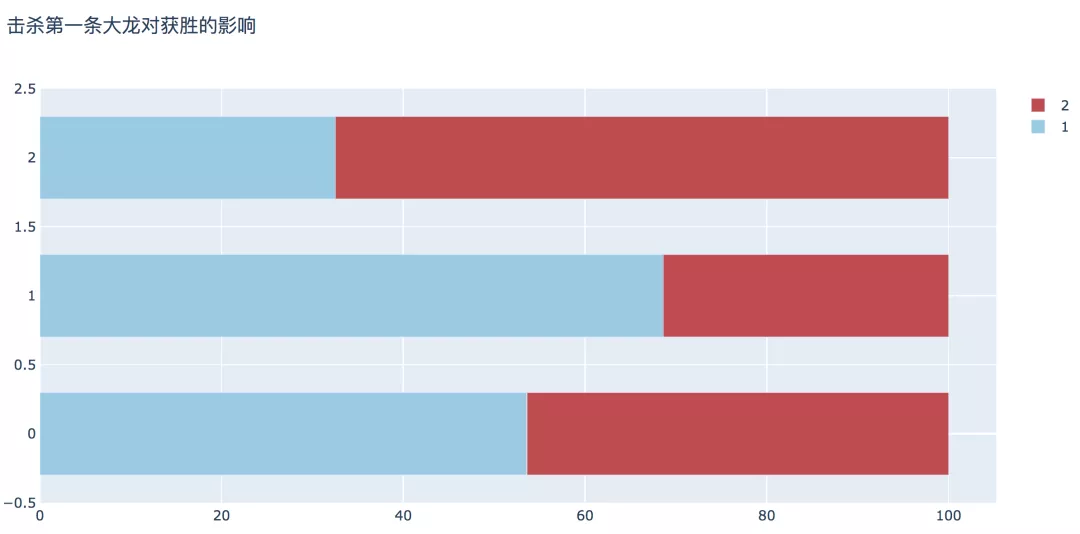```plot_bar_horizontal(input_col='firstDragon',
target_col='winner',
title_name='击杀第一条大龙对获胜的影响') ```

8. 击杀第一条峡谷先锋对获胜的影响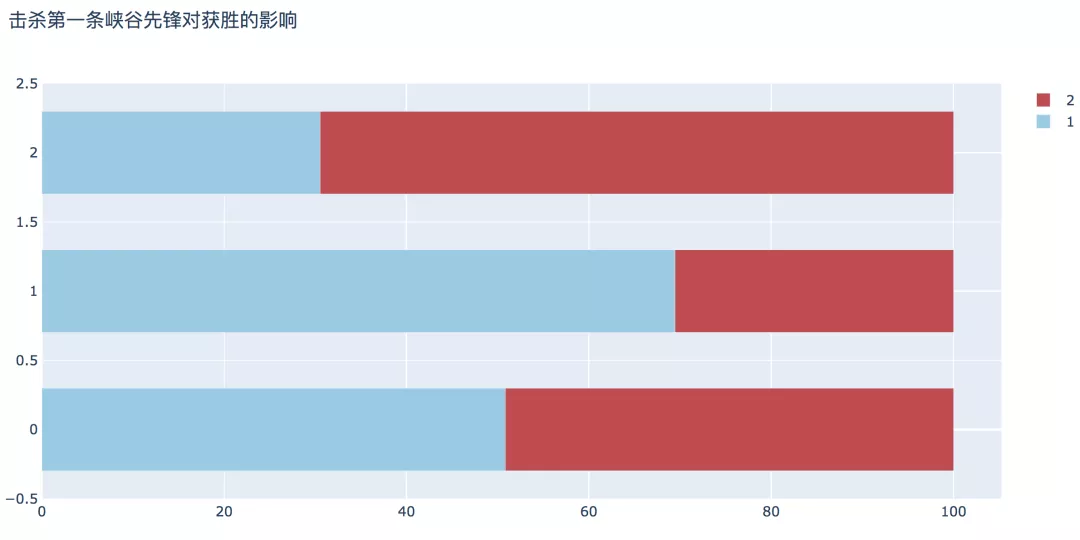```plot_bar_horizontal(input_col='firstRiftHerald',
target_col='winner',
title_name='击杀第一条峡谷先锋对获胜的影响')
```

9. 摧毁防御塔数对获胜影响```plot_bar_vertical(input_col='t1_towerKills',
target_col='winner',
title_name='摧毁防御塔数对获胜影响')  ```

10. 摧毁水晶数对获胜影响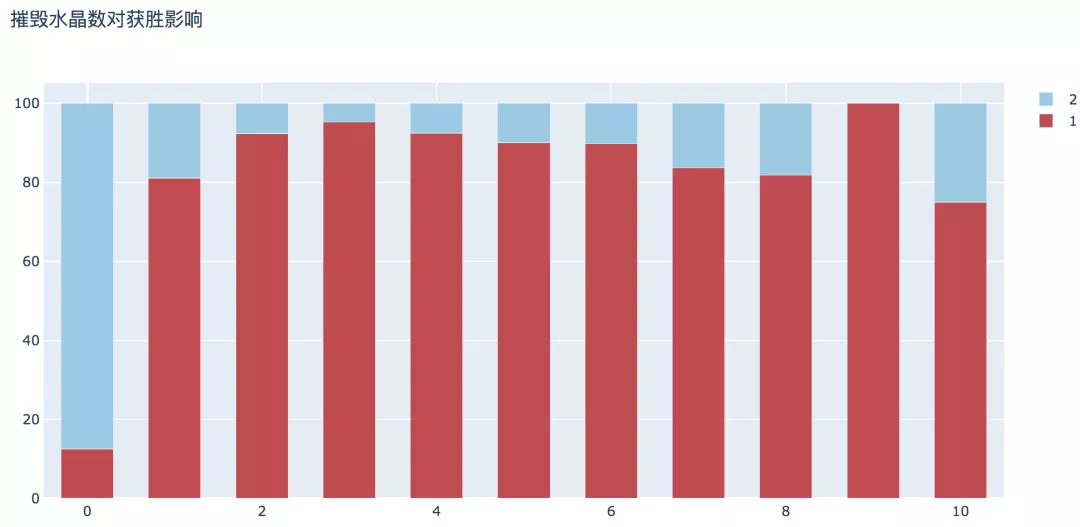```plot_bar_vertical(input_col='t1_inhibitorKills',
target_col='winner',
title_name='摧毁水晶数对获胜影响')  ```

11. 击杀男爵数对获胜影响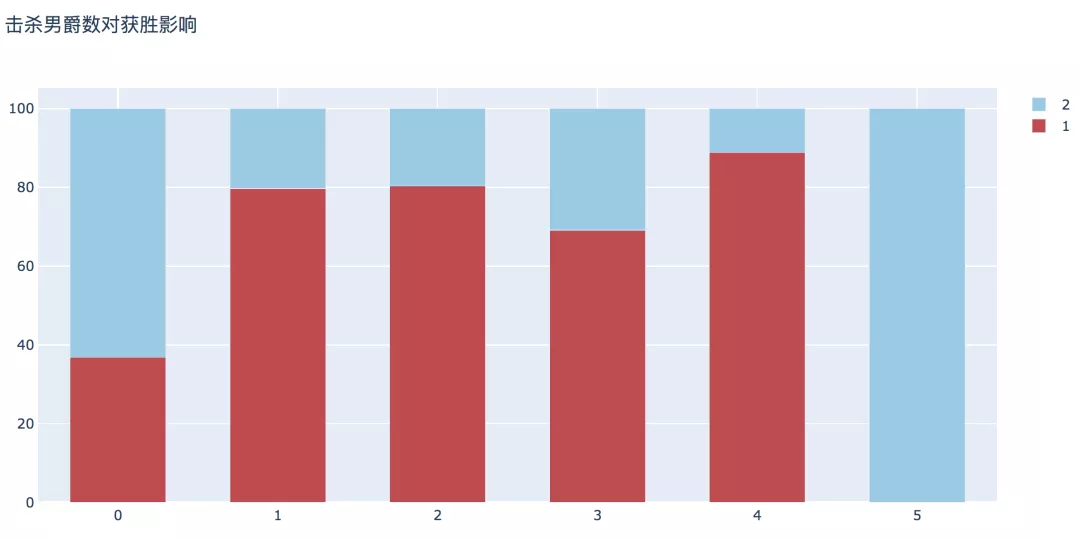```plot_bar_vertical(input_col='t1_baronKills',
target_col='winner',
title_name='击杀男爵数对获胜影响')  ```

12. 击杀大龙数对获胜影响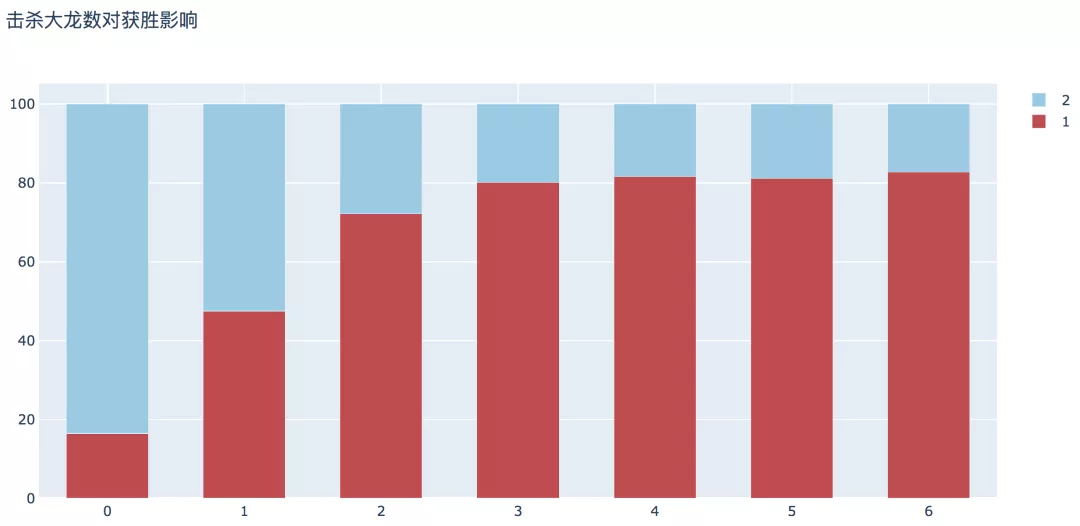```plot_bar_vertical(input_col='t1_dragonKills',
target_col='winner',
title_name='击杀大龙数对获胜影响') ```

05、数据建模

```# 删除时间少于15分钟和分类数较少的记录
df = df[(df['gameDuration'] >= 900) & (df['t1_baronKills'] != 5)]
print(df.shape) ```
`(50180, 62)`
```# 筛选建模变量
df_model = df[['winner', 'firstBlood', 'firstTower', 'firstInhibitor', 'firstBaron',
'firstDragon', 'firstRiftHerald', 't1_towerKills', 't1_inhibitorKills','t1_baronKills',
't1_dragonKills', 't2_towerKills', 't2_inhibitorKills', 't2_baronKills', 't2_dragonKills'
]]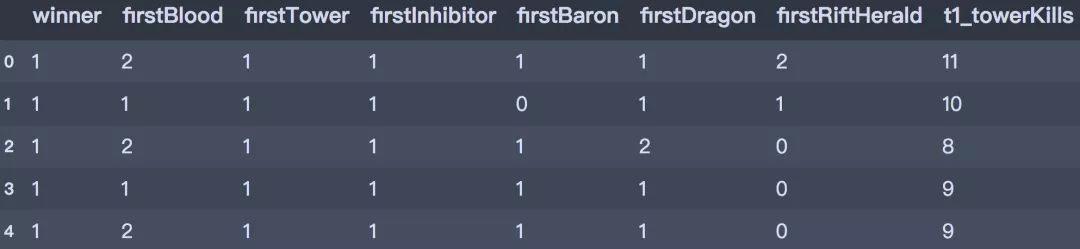```# 划分训练集和测试集
x = df_model.drop('winner', axis=1)
y = df_model['winner']

X_train, X_test, y_train, y_test = train_test_split(x, y, test_size=0.2, stratify=y, random_state=0)
print(X_train.shape, X_test.shape, y_train.shape, y_test.shape) ```
`(40144, 14) (10036, 14) (40144,) (10036,)`

```# 参数
parameters = {
'splitter': ('best', 'random'),
'criterion':('gini', 'entropy'),
'max_depth':[*range(1, 20, 2)],
}

# 建立模型
clf = DecisionTreeClassifier(random_state=0)
GS = GridSearchCV(clf, parameters, cv=10)
GS.fit(X_train, y_train)  ```
```GridSearchCV(cv=10, estimator=DecisionTreeClassifier(random_state=0),
param_grid={'criterion': ('gini', 'entropy'),
'max_depth': [1, 3, 5, 7, 9, 11, 13, 15, 17, 19],
'splitter': ('best', 'random')})
```
```# 输出最佳得分
print("best score: ", GS.best_score_)
print("best param: ", GS.best_params_) ```
```best score:  0.9770077890521407
best param:  {'criterion': 'gini', 'max_depth': 7, 'splitter': 'best'}```
```# 最佳模型
best_clf = DecisionTreeClassifier(criterion="gini", max_depth=7, splitter="best")
best_clf.fit(X_train,y_train)
print("score:", best_clf.score(X_test,y_test))   ```
`score: 0.9799721004384216  `

```# 输出分类报告
y_pred = best_clf.predict(X_test)
cm = confusion_matrix(y_test, y_pred)
cr = classification_report(y_test, y_pred)
print('Classification report : n', cr)  ```
```Classification report :
precision    recall  f1-score   support

1       0.98      0.98      0.98      5077
2       0.98      0.98      0.98      4959

accuracy                           0.98     10036
macro avg       0.98      0.98      0.98     10036
weighted avg       0.98      0.98      0.98     10036```
```# 热力图
g1 = sns.heatmap(cm, annot=True, fmt=".1f", cmap="flag", linewidths=0.2, cbar=False)
g1.set_ylabel('y_true', fontdict={'fontsize': 15})
g1.set_xlabel('y_pred', fontdict={'fontsize': 15})
g1.set_title('confusion_matrix', fontdict={'fontsize': 15})  ```
`Text(0.5, 1, 'confusion_matrix')````# 输出属性重要性
imp = pd.DataFrame(list(zip(X_train.columns, best_clf.feature_importances_)))
imp.columns = ['columns', 'importances']
imp = imp.sort_values('importances', ascending=False)
imp ```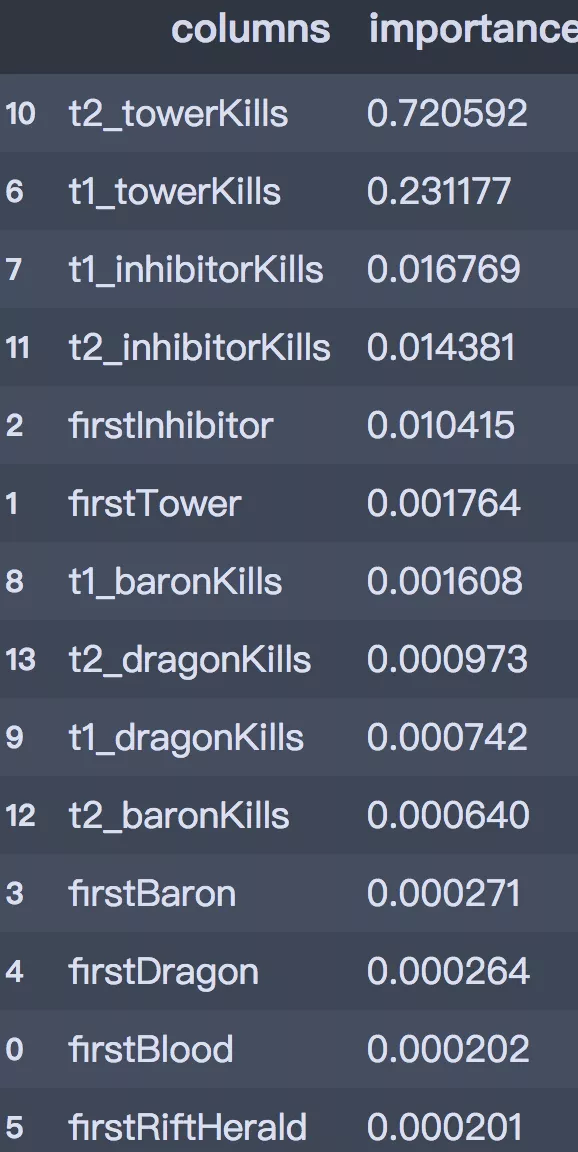```# 可视化
import graphviz
from sklearn import tree

dot_data = tree.export_graphviz(decision_tree=best_clf, max_depth=3,
out_file=None,
feature_names=X_train.columns,
class_names=['1', '2'],
filled=True,
rounded=True
)
graph = graphviz.Source(dot_data)
graph```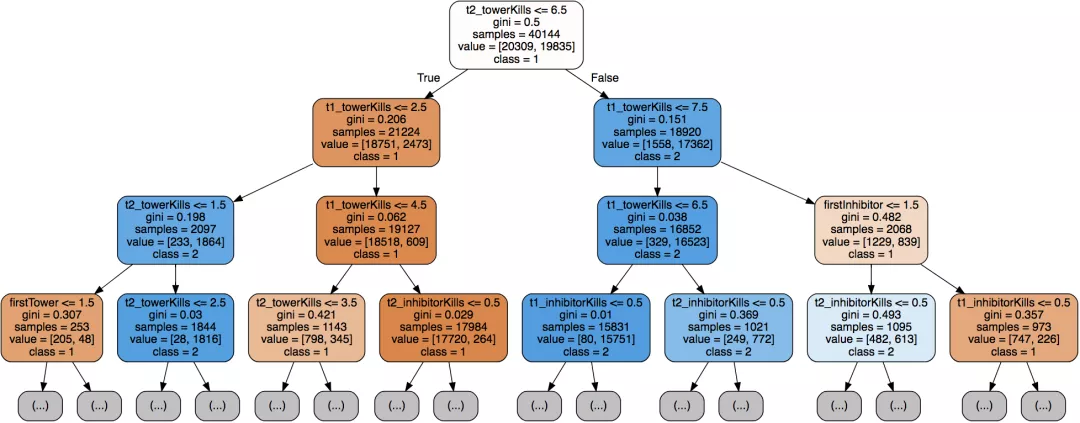06、模型预测

```# 新数据
new_data = [[1, 1, 2, 1, 1, 1, 10, 2, 1, 4, 7, 2, 1, 1]]
c = best_clf.predict_proba(new_data).reshape(-1, 1)
print("winner is :" , best_clf.predict(x1))
print("First team win probability is % ", list(c * 100),
"nSecond team win probability is %:",list(c * 100)) ```
```winner is : 
First team win probability is %  [89.87341772151899]
Second team win probability is %: [10.126582278481013]```

——热门课程推荐：

OK